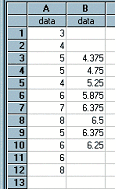﻿ MPA(column,cyclelen)

# MPA(column,cyclelen)

Top  Previous  Next
 Calculates the moving point average, or centred moving point average, for the data in column with a cycle length of cyclelen.   The MPA function automatically centres the moving point average if cyclelen is even.   The data in column B of the example has been generated using MPA(A,4)Note:  If you type decimals using a comma (eg 3,2) you should enter this formula as MPA(A ; 4) - using the ; as a separator